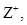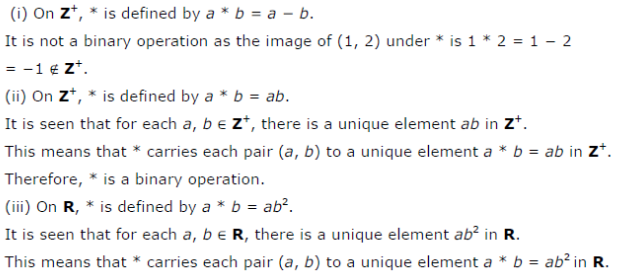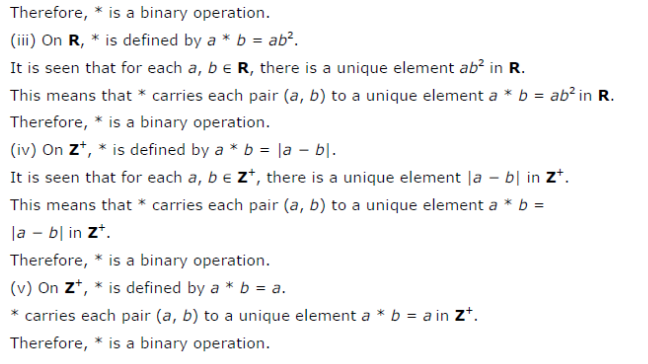# Determine whether or not each of the definition of * given below gives a binary operation

Determine whether or not each of the definition of * given below gives a binary operation. In the event that * is not a binary operation, given justification for this.
(i) Ondefine * by a * b = a - b
(ii) Ondefine * by a * b = ab
(iii) On R, define * by a * b = ab2
(iv) Ondefine * by a * b = | a - b |
(v) Ondefine * by a * b = a**